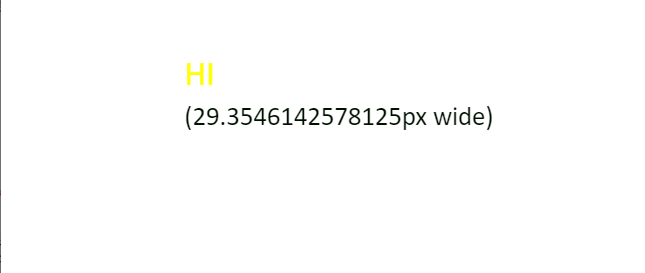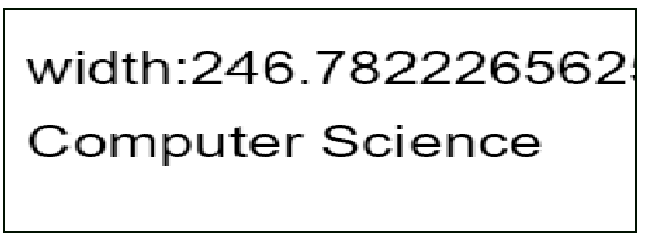# How to find the height of a text in HTML canvas using JavaScript ?

• Difficulty Level : Medium
• Last Updated : 25 Dec, 2020

In this article, we will find the height of the text canvas using JavaScript.

Approach: In the following example, the height attribute of the HTML canvas is used. First set the font in pt to set the height.

`context.font = '26pt Calibri';`

Then the current text is aligned in the center by using values ‘middle’ and color ‘yellow’.

```context.textAlign = 'middle';
context.fillStyle = 'yellow';```

Then, check the width of the text using the measureText() method before writing to the canvas. Finally, the text is written on the canvas using the fillText() method.

Example 1:

## HTML

 ` ` `<``html``> ` ` `  `<``head``> ` `    ``<``style``> ` `        ``body { ` `            ``margin: 0px; ` `            ``padding: 0px; ` `        ``} ` `    `` ` ` ` ` `  `<``body``> ` `    ``<``canvas` `id``=``"myCanvas"` `width``=``"550"` `height``=``"200"``> ` `    `` ` `    ``<``script``> ` `        ``var canvas = document.getElementById('myCanvas'); ` `        ``var context = canvas.getContext('2d'); ` `        ``var x = canvas.width / 3; ` `        ``var y = canvas.height / 2 - 15; ` `        ``var text = 'HI '; ` ` `  `        ``// Set the font to set the height ` `        ``context.font = '26pt Calibri'; ` `        ``context.textAlign = 'middle'; ` `        ``context.fillStyle = 'yellow'; ` `        ``context.fillText(text, x, y); ` `        ``context.font = '20pt Calibri'; ` ` `  `        ``// Check the width of the text  ` `        ``var metrics = context.measureText(text); ` `        ``var width = metrics.width; ` ` `  `        ``// Text is aligned in the left       ` `        ``context.textAlign = 'left'; ` `        ``context.fillStyle = '#010'; ` `         `  `        ``context.fillText('(' + width  ` `            ``+ 'px wide)', x, y + 40); ` `    `` ` ` ` ` `  ` `

Output:Approach: First get the 2d drawing context of the canvas by using getContext() method. Set the font and the text string. Then write the text with x and y co-ordinates using fillText() method as given below.

Example 2:

## HTML

 ` ` `<``html``> ` ` `  `<``body``> ` `    ``<``canvas` `id``=``"Canvas"` `width``=``"300"` `height``=``"150"`  `        ``style``=``"border:1px solid #010;"``> ` `        ``Your browser isn't supporting  ` `        ``HTML5 canvas tag. ` `    `` ` ` `  `    ``<``script``> ` `        ``var c = document.getElementById("Canvas"); ` `        ``var x = c.getContext("2d"); ` `        ``x.font = "30px Arial"; ` `        ``var txt = "Computer Science" ` `        ``x.fillText("width:"  ` `            ``+ x.measureText(txt).width, 10, 50); ` `        ``x.fillText(txt, 10, 100); ` `    `` ` ` ` ` `  ` `

Output:My Personal Notes arrow_drop_up
Recommended Articles
Page :